# One green

In the container are 45 white and 15 balls. We randomly select 5 balls. What is the probability that it will be a maximum one green?

p =  0.6329

### Step-by-step explanation:

C_{{ 5}}(60) = \dbinom{ 60}{ 5} = \dfrac{ 60! }{ 5!(60-5)!} = \dfrac{ 60 \cdot 59 \cdot 58 \cdot 57 \cdot 56 } { 5 \cdot 4 \cdot 3 \cdot 2 \cdot 1 } = 5461512 \ \\ C_{{ 0}}(15) = \dbinom{ 15}{ 0} = \dfrac{ 15! }{ 0!(15-0)!} = \dfrac{ 1 } { 1 } = 1 \ \\ C_{{ 1}}(15) = \dbinom{ 15}{ 1} = \dfrac{ 15! }{ 1!(15-1)!} = \dfrac{ 15 } { 1 } = 15 \ \\ C_{{ 5}}(45) = \dbinom{ 45}{ 5} = \dfrac{ 45! }{ 5!(45-5)!} = \dfrac{ 45 \cdot 44 \cdot 43 \cdot 42 \cdot 41 } { 5 \cdot 4 \cdot 3 \cdot 2 \cdot 1 } = 1221759 \ \\ C_{{ 4}}(45) = \dbinom{ 45}{ 4} = \dfrac{ 45! }{ 4!(45-4)!} = \dfrac{ 45 \cdot 44 \cdot 43 \cdot 42 } { 4 \cdot 3 \cdot 2 \cdot 1 } = 148995 \ \\ \ \\ p = \dfrac\dbinom{ {15}{ 0}\dbinom{ 45}{ 5} + \dbinom{ 15}{ 1}\dbinom{ 45}{ 4} }\dbinom{ { 45+15}{ 5} } = 0.6329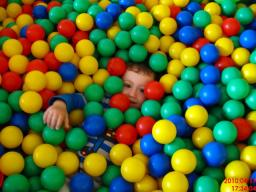Did you find an error or inaccuracy? Feel free to write us. Thank you!Tips to related online calculators
Would you like to compute count of combinations?

## Related math problems and questions:

• BallsThe urn is 8 white and 6 black balls. We pull 4 randomly balls. What is the probability that among them will be two white?
• White and black ballsThere are 7 white and 3 black balls in an opaque pocket. The balls are the same size. a) Randomly pull out one ball. What is the probability that it will be white? We pull out one ball, see what color it is and return it to the pocket. Then we pull out th
• Round destiny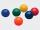There are 5 white and 10 red balls in the destiny. 4 balls will be drawn at random. What is the probability of the event "at least 2 spheres are white"?
• Disco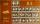At the disco goes 12 boys and 15 girls. In how many ways can we select four dancing couples?
• BallsFrom the urn in which are 7 white balls and 17 red, gradually drag 3-times without replacement. What is the probability that pulls balls are in order: red red red?
• Red ballsIn the bag there are 3 red, 12 blue and 8 green balls. How many red balls we must be attached to the bag if we want the probability of pulling out the red balls was 20%?
• Balls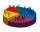We have n identical balls (numbered 1-n) is selected without replacement. Determine 1) The probability that at least one tensile strength number coincides with the number of balls? 2) Determine the mean and variance of the number of balls, which coincides
• Green - Red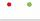We have 5 bags. Each consist one green and 2 red balls. From each we pull just one ball. What is the probability that we doesn't pull any green ball?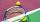The children of the tennis school received 64 white and 48 yellow balls from the sponsor. When asked about how many balls they could take, they answered: "You have so many that none of you will have more than ten balls, and all will have the same number o
• Three colors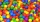Find the probability that 3 balls of the same color will be drawn from fate with 10 white, 10 red, and 10 blue balls.
• Statistics quizFill in the missing word 1. in a data set, the mean, median and mode are measured of ________________ 2. "The manipulation of variables under controlled conditions"is the data collection method known as______________ 3. in a normal distribution, the area
• CardsFrom a set of 32 cards, we randomly pull out three cards. What is the probability that it will be seven kings and ace?
• Raffle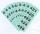In the pool is numbers from 1 to 115. What is the probability that a randomly selected number is not a prime number?
• First class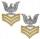The shipment contains 40 items. 36 are first grade, 4 are defective. How many ways can select 5 items, so that it is no more than one defective?
• Speed of Slovakian trainsRudolf decided to take the train from the station 'Ostratice' to 'Horné Ozorovce'. In the train timetables found train Os 5409 : km 0 Chynorany 15:17 5 Ostratice 15:23 15:23 8 Rybany 15:27 15:27 10 Dolné Naštice 15:31 15:31 14 Bánovce nad Bebravou 15:35 1
• Competition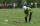15 boys and 10 girls are in the class. On school competition of them is selected 6-member team composed of 4 boys and 2 girls. How many ways can we select students?
• Bulbs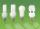In the box are 6 bulbs with power 75 W, 14 bulbs with power 40 W and 15 with 60 W. Calculate the probability that a randomly selected bulb is: